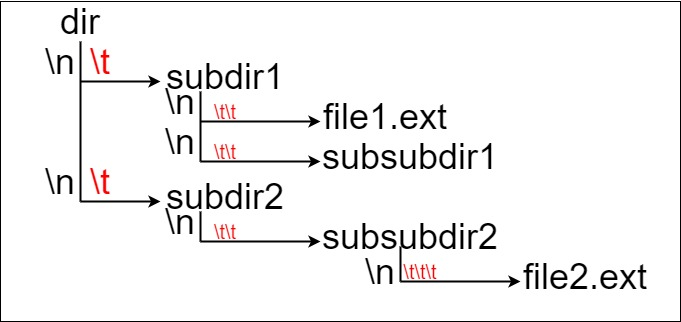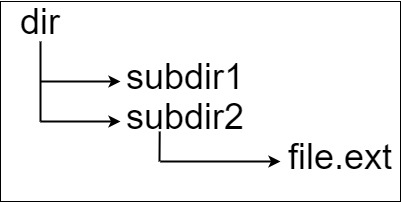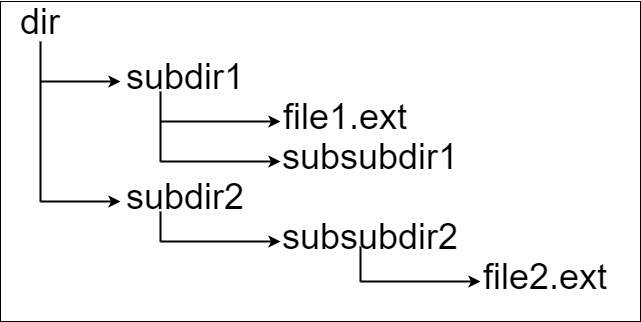388. Longest Absolute File Path

Suppose we have a file system that stores both files and directories. An example of one system is represented in the following picture:Here, we have `dir` as the only directory in the root. `dir` contains two subdirectories, `subdir1` and `subdir2`. `subdir1` contains a file `file1.ext` and subdirectory `subsubdir1`. `subdir2` contains a subdirectory `subsubdir2`, which contains a file `file2.ext`.

In text form, it looks like this (with ⟶ representing the tab character):

```dir
⟶ subdir1
⟶ ⟶ file1.ext
⟶ ⟶ subsubdir1
⟶ subdir2
⟶ ⟶ subsubdir2
⟶ ⟶ ⟶ file2.ext
```

If we were to write this representation in code, it will look like this: `"dir\n\tsubdir1\n\t\tfile1.ext\n\t\tsubsubdir1\n\tsubdir2\n\t\tsubsubdir2\n\t\t\tfile2.ext"`. Note that the `'\n'` and `'\t'` are the new-line and tab characters.

Every file and directory has a unique absolute path in the file system, which is the order of directories that must be opened to reach the file/directory itself, all concatenated by `'/'s`. Using the above example, the absolute path to `file2.ext` is `"dir/subdir2/subsubdir2/file2.ext"`. Each directory name consists of letters, digits, and/or spaces. Each file name is of the form `name.extension`, where `name` and `extension` consist of letters, digits, and/or spaces.

Given a string `input` representing the file system in the explained format, return the length of the longest absolute path to a file in the abstracted file system. If there is no file in the system, return `0`.

Example 1:```Input: input = "dir\n\tsubdir1\n\tsubdir2\n\t\tfile.ext"
Output: 20
Explanation: We have only one file, and the absolute path is "dir/subdir2/file.ext" of length 20.
```

Example 2:```Input: input = "dir\n\tsubdir1\n\t\tfile1.ext\n\t\tsubsubdir1\n\tsubdir2\n\t\tsubsubdir2\n\t\t\tfile2.ext"
Output: 32
Explanation: We have two files:
"dir/subdir1/file1.ext" of length 21
"dir/subdir2/subsubdir2/file2.ext" of length 32.
We return 32 since it is the longest absolute path to a file.
```

Example 3:

```Input: input = "a"
Output: 0
Explanation: We do not have any files, just a single directory named "a".
```

Example 4:

```Input: input = "file1.txt\nfile2.txt\nlongfile.txt"
Output: 12
Explanation: There are 3 files at the root directory.
Since the absolute path for anything at the root directory is just the name itself, the answer is "longfile.txt" with length 12.
```

Constraints:

• `1 <= input.length <= 104`
• `input` may contain lowercase or uppercase English letters, a new line character `'\n'`, a tab character `'\t'`, a dot `'.'`, a space `' '`, and digits.

388. Longest Absolute File Path
``````struct Solution;

struct Folder {
level: usize,
length: usize,
}

impl Solution {
fn length_longest_path(input: String) -> i32 {
let mut stack: Vec<Folder> = vec![];
let mut folder_length = 0;
let mut max = 0;
for line in input.split('\n') {
let s = line.chars();
let mut level: usize = 0;
let mut is_folder = true;
for c in s {
match c {
'\t' => {
level += 1;
}
'.' => {
is_folder = false;
break;
}
_ => {}
}
}
let name = line[level..].to_string();
while let Some(top) = stack.pop() {
if top.level >= level {
folder_length -= top.length;
} else {
stack.push(top);
break;
}
}
if is_folder {
let length = name.len() + 1;
let folder = Folder { level, length };
stack.push(folder);
folder_length += length;
} else {
max = max.max(name.len() + folder_length);
}
}
max as i32
}
}

#[test]
fn test() {
let input =
"dir\n\tsubdir1\n\t\tfile1.ext\n\t\tsubsubdir1\n\tsubdir2\n\t\tsubsubdir2\n\t\t\tfile2.ext"
.to_string();
let res = 32;
assert_eq!(Solution::length_longest_path(input), res);
}
``````